Next: More Matrix Eigenvalue Theory Up: Coupled Oscillations Previous: Equilibrium State

Stability Equations

It is evident that if our system is initialized in some equilibrium state, with all of the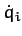set to zero, then it will remain in this state for ever. But what happens if the system is slightly perturbed from the equilibrium state?

Let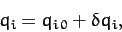(774)

for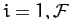, where the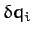are small. To lowest order in, the kinetic energy (770) can be written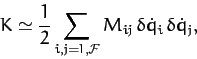(775)

where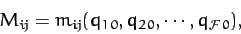(776)

and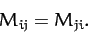(777)

Note that the weights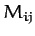in the quadratic form (775) are now constants.

Taylor expanding the potential energy function about the equilibrium state, up to second-order in the, we obtain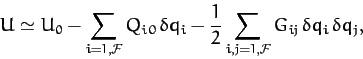(778)

where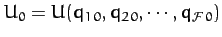, the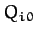are specified in Equation (773), and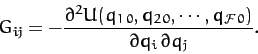(779)

Now, we can set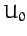to zero without loss of generality. Moreover, according to Equation (773), theare all zero. Hence, the expression (778) reduces to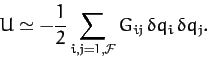(780)

Note that, since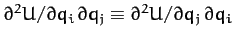, the constants weights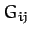in the above quadratic form are invariant under interchange of the indicesand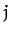: i.e.,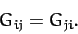(781)

Withand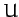specified by the quadratic forms (775) and (780), respectively, Lagrange's equations of motion (772) reduce to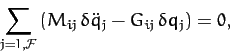(782)

for. Note that the above coupled differential equations are linear in the. It follows that the solutions are superposable. Let us search for solutions of the above equations in which all of the perturbed coordinateshave a common time variation of the form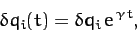(783)

for. Now, Equations (782) are a set of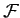second-order differential equations. Hence, the most general solution contains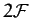arbitrary constants of integration. Thus, if we can find sufficient independent solutions of the form (783) to Equations (782) that the superposition of these solutions containsarbitrary constants then we can be sure that we have found the most general solution. Equations (782) and (783) yield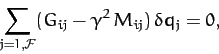(784)

which can be written more succinctly as a matrix equation: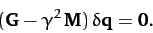(785)

Here,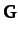is the real [see Equation (779)] symmetric [see Equation (781)]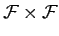matrix of thevalues. Furthermore,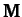is the real [see Equation (770)] symmetric [see Equation (777)]matrix of thevalues. Finally,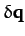is the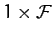vector of thevalues, and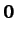is a null vector.Next: More Matrix Eigenvalue Theory Up: Coupled Oscillations Previous: Equilibrium State
Richard Fitzpatrick 2011-03-31